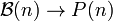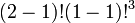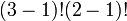# Combinatorics of symmetric group:S5

Jump to: navigation, search

## Contents

This article gives specific information, namely, combinatorics, about a particular group, namely: symmetric group:S5.
View combinatorics of particular groups | View other specific information about symmetric group:S5

This page discusses some of the combinatorics associated with symmetric group:S5, that relies specifically on viewing it as a symmetric group on a finite set.

## Partitions, subset partitions, and cycle decompositions

Denote by$\mathcal{B}(n)$ the set of all unordered set partitions of$\{ 1,2,\dots,n\}$ into subsets and by$P(n)$ the set of unordered integer partitions of$n$. There are natural combinatorial maps:$S_n \to \mathcal{B}(n) \to P(n)$

where the first map sends a permutation to the subset partition induced by its cycle decomposition, which is equivalently the decomposition into orbits for the action of the cyclic subgroup generated by that permutation on$\{ 1,2,\dots,n\}$. The second map sends a subset partition to the partition of$n$ given by the sizes of the parts. The composite of the two maps is termed the cycle type, and classifies conjugacy classes in$S_n$, because cycle type determines conjugacy class.

Further, if we define actions as follows:

•$S_n$ acts on itself by conjugation
•$S_n$ acts on$\mathcal{B}(n)$ by moving around the elements and hence changing the subsets
•$S_n$ acts on$P(n)$ trivially

then the maps above are$S_n$-equivariant, i.e., they commute with the$S_n$-action. Moreover, the action on$\mathcal{B}(n)$ is transitive on each fiber above$P(n)$ and the action on$S_n$ is transitive on each fiber above the composite map to$P(n)$. In particular, for two elements of$\mathcal{B}(n)$ that map to the same element of$P(n)$, the fibers above them in$S_n$ have the same size.

There are formulas for calculating the sizes of the fibers at each level.

In our case$n = 5$:

Partition Partition in grouped form Formula calculating size of fiber for$\mathcal{B}(n) \to P(n)$ Size of fiber for$\mathcal{B}(n) \to P(n)$ Formula calculating size of fiber for$S_n \to \mathcal{B}(n)$ Size of fiber for$S_n \to \mathcal{B}(n)$ Formula calculating size of fiber for$S_n \to P(n)$, which is precisely the conjugacy class size for that cycle type (product of previous two formulas) Size of fiber for$S_n \to P(n)$, which is precisely the conjugacy class size for that cycle type (product of previous two sizes)
1 + 1 + 1 + 1 + 1 1 (5 times)$\frac{5!}{(1!)^55!}$ 1$(1 - 1)!^5$ 1$\frac{5!}{1^55!}$ 1
2 + 1 + 1 + 1 2 (1 time), 1 (3 times)$\frac{5!}{(2!)^11!(1!)^3(3!)}$ 10$(2 - 1)!(1 - 1)!^3$ 1$\frac{5!}{2^11!(1)^33!}$ 10
3 + 1 + 1 3 (1 time), 1 (2 times)$\frac{5!}{(3!)^11!(1!)^22!}$ 10$(3 - 1)!(1 - 1)!^2$ 2$\frac{5!}{3^11!(1)^22!}$ 20
2 + 2 + 1 2 (2 times), 1 (1 time)$\frac{5!}{(2!)^22!(1!)^11!}$ 15$(2 - 1)!^2(1 - 1)!$ 1$\frac{5!}{2^22!1^11!}$ 15
4 + 1 4 (1 time), 1 (1 time)$\frac{5!}{(4!)^11!(1!)^11!}$ 5$(4 - 1)!(1 - 1)!$ 6$\frac{5!}{4^11!1^11!}$ 30
3 + 2 3 (1 time), 2 (1 time)$\frac{5!}{(3!)^11!(2!)^11!}$ 10$(3 - 1)!(2 - 1)!$ 2$\frac{5!}{3^11!2^11!}$ 20
5 5 (1 time)$\frac{5!}{(5!)^11!}$ 1$(5 - 1)!$ 24$\frac{5!}{5^11!}$ 24
Total (7 rows -- number of rows equals number of unordered integer partitions of 5) -- -- 52 (equals the size of$\mathcal{B}(n)$, termed the Bell number, at$n = 5$) -- 37 (see Oeis:A107107) -- 120 (equals 5!, the size of the symmetric group)